# Mathematical Modelling of MHD Natural Convection in a Linearly Heated Porous Cavity

Mathematical Modelling of MHD Natural Convection in a Linearly Heated Porous Cavity
Department of Basic and Applied Science, NIT Arunachal Pradesh, Yupia 791112, India

Corresponding Author Email:
vanav@nitap.ac.in
Page:
149-157
|
DOI:
https://doi.org/10.18280/mmep.080119
26 November 2020
|
Accepted:
3 February 2021
|
Published:
28 February 2021
| Citation

OPEN ACCESS

Abstract:

A linear increase in thermal boundaries towards the bottom of the porous cavity is considered for numerical flow analysis on MHD natural convection. The two-dimensional square shaped cavity is filled with the Cu-water nanofluid. The dimensionless equations are considered to interpret the fluid and heat flow inside the cavity with respect to the desired boundaries. The governing equations are solved using the finite difference techniques. The relevant dimensionless parameters used in the present study are Rayleigh number, Darcy number, solid volume fraction of the nanoparticles and Hartmann number to obtain the flow fields. Heatline flows picturization techniques involved in the study analyze the heat flow inside the cavity. As the Rayleigh number and Darcy number increases, an increase in streamlines flow velocity and convection heat transfer is observed. Convective heat transfer is interrupted by increasing the applied magnetic field effects. An improvement in the heat transfer is noticed by increasing the solid volume fraction of the particles.

Keywords:

cavity, porous, linearly heated, heatlines, natural convection, magnetic field

1. Introduction

The importance of flow analysis in a porous enclosure due to application in various fields such as modeling of phase change problems, solidification, crystal growth, contaminant transports, fluid flows on the underground, underground spread of pollutants, geophysical problems, etc. The applications and importance of convection in porous media are found in the literature [1-3].

Attention is given to the study of convective flow and heat transfer in rectangular shaped cavities/enclosure by various authors: Oztop and Abu-Nada  analyzed the variation of Nusselt number obtained due to natural convection for various height and position of the heater in a nanofluid filled square cavity. Sathiyamoorthy and Chamkha  discussed the effects of inclination on the applied magnetic field for a linearly heated, natural convective cavity filled with an electrically conducting fluid. Sivasankaran et al.  investigated the combined effect of convection and magnetic field in a lid-driven cavity heated non-uniformly along the vertical walls. Al-Salem et al.  studied the convection flow in the magneto-lid-driven cavity due to linearly varying temperature boundaries. Nasrin and Alim  focused on analyzing the effects of Soret and Dufort on natural convection in a double-diffusive cavity. Sathiyamoorthy and Chamkha  set a thermal boundary profile of constant heating at the bottom wall and linearly heating at the sidewalls of a square cavity with a thin partition inside. The above study is conducted to explain the fluid flow and heat transfer that occurs due to natural convection. Ahrara et al.  simulated the flow that occurs in the Cu-water nanofluid from the combinations of natural convection, magnetic fields and its inclination effects on a cavity with a circular cylinder inside. Mahmoodi et al.  presented a work to demonstrate the water-Al2O3 nanofluid flow behavior on the square cavity by different combinations of linearly cooled/heated-left/right walls due to natural convection. Tayebi and Chamkha  studied the flow characteristics generated by various running fluids such as hybrid nanofluid, normal nanofluid and a base fluid using buoyancy-driven effects. Alam et al.  analyzed the convection heat transfer, which occurs due to the heated base of the cavity, filled with nanofluids and obstacles present in the center of the cavity subjected to the applied magnetic field effect. Triveni and Panua  appraised the heat transfer generated by nanofluids such as Al2O3, TiO2, CuO – H2O due to the effect of natural convection on the caterpillar-shaped enclosure. Chaabane and Jemni  studied the MHD natural convection on linearly heated cavities. Giwa et al.  reviewed the overall influence of magnetic field effect on square cavities of various combinations of thermal and velocity boundaries. Al-Kouz et al.  studied the free convective rarefied gas flow in an inclined and oblique wave cavity. The importance of incorporating the study of heatlines in the heat transfer analysis process is discussed in the publications [18-21]. Oztop et al.  briefly discussed the natural convective heat flow that occurs by the linearly varying temperature on a left side wall of the square cavity, containing CuO-water nanofluid. Natural convective heat transfer occurs in a prismatic-shaped cavity using heatlines are discussed by Ahmed et al.  and Alam et al. . Alsabery et al.  have clearly demonstrated the heat flow, which occurs in the solid phase by conduction and nanofluid phase due to the sinusoidal thermal boundaries by convection.

Flow structures formed by the aspect ratios of the porous rectangular cavities have been discussed by Walker and Homsy  and by Prasad and Kulacki . Mahmud and Fraser  discussed the magnetohydrodynamic effects on natural convective porous cavity by considering Darcy’s law. Here, the flow is suppressed and a reduction in the average Nusselt number for applying the magnetic field is noted. Sathiyamoorthy et al.  simulated the natural convective flow caused by the linearly heated vertical boundaries and evenly heated bottom boundary using Galerkin FEM. In their study, two vertically symmetric circulations are noted at a lower Darcy number and multiple circulations with clockwise and anticlockwise directions are found at higher Darcy numbers. Varol et al.  investigated the effects of sinusoidally bounded temperature distribution at the bottom wall on the porous cavity during natural convection. Basak et al.  approached the heat transfer analysis by using heatlines on the lid-driven porous cavity with mixed thermal wall boundaries of evenly, sinusoidally and linearly heated. The heatlines study shows an increase in the convection heat transfer due to an increase in the Rayleigh number and the Darcy number. Ramakrishna et al.  studied the heatlines flow generated by the action of combined boundaries of linear and sinusoidal heated walls on natural convective porous cavities. Kasaeian et al.  examined the fluid and heat flow by nanofluid within the porous enclosure of different shapes and orientations. Abdulkadhim et al.  investigated the natural convective flow effects on the partially heated and cooled porous cavity. Al-Srayyih et al.  considered a linearly heated cavity of two partitions with a nanofluid and with a porous layer containing nanofluid respectively. The study includes the heat transfer and flow field due to natural convection by changing the orientation of the partition. Vanav et al.  investigated the flow by a non-uniform heated cavity of porous media due to natural convection. Belhadj et al.  analyzed the convection on porous cavity (Darcy-Brinkman-Forcheimer model). The study shows an increase in the average Nusselt number and streamlines velocity as the Darcy number increases. Bouafia et al.  focused on analyzing the natural convective flow generated by applied constant temperature on the vertical walls (hatched portion) of a porous cavity. Jino and Vanav [35, 36] involved in the investigation of natural convection on a porous cavity (Carman-Kozeny equation model) by considering the magnetic field and various thermal boundaries.

In this study, flow for combined boundaries of linearly heated and constantly heated porous cavity on magnetic field effect and natural convection is considered. The computations are performed for the proposed non-dimensional parameters and the results are discussed using Nusselt numbers, streamlines, isotherms and heatlines.

2. Mathematical Modelling

A 2-D mathematical model considers a square-shaped porous cavity with each side of dimension, H. Each side walls have zero velocity and thermally constant temperature of Th at the bottom, linearly varying temperature of $T_{h}-\left(T_{h}-T_{l}\right) y / H$ at the vertical walls and a thermally insulated top wall. Here Th denotes the higher temperature and Tl denotes the lower temperature as illustrated in Figure 1. Inside the cavity, Cu nanoparticles are dispersed in the water under a porous medium assuming the flow is laminar, Newtonian and incompressible. Considering Boussinesq approximation and neglecting other effects such as radiation, viscous dissipation, joule heating and heat generation/absorption. Applied magnetic fields are taken into the effects, which act in the horizontal direction. The temperature of the nanofluid flow and a porous bed is in thermal equilibrium. A fluid in a porous bed is designed using the Darcy–Brinkman model with neglecting the inertia effect that occurs due to the Forchheimers term in the momentum equations .

## 1.png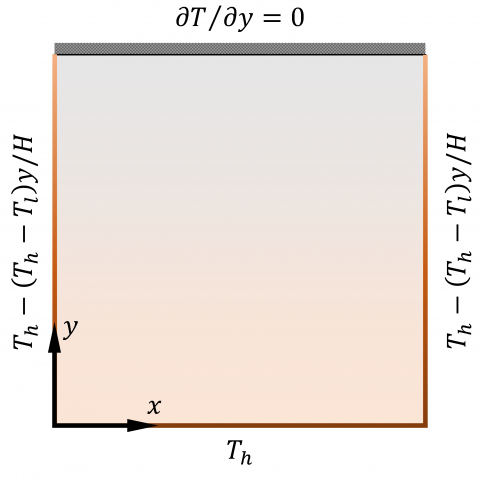Figure 1. Physical geometry of the problem

With the above assumptions, a set of governing equations are considered to describe the MHD natural convective flow of nanofluid inside a porous cavity. The flow field equations using the combination of continuity, momentum and energy equations as,

$\frac{\partial u}{\partial x}+\frac{\partial v}{\partial y}=0$      (1)

$\rho_{n}\left[u \frac{\partial u}{\partial x}+v \frac{\partial u}{\partial y}\right]=\mu_{n}\left(\nabla^{2} \cdot \boldsymbol{u}\right)-\frac{\partial p}{\partial x}-u \frac{\mu_{n}}{K}$      (2)

\begin{aligned} \rho_{n}\left[u \frac{\partial v}{\partial x}+v \frac{\partial v}{\partial y}\right]=& \mu_{n}\left(\nabla^{2} \cdot \boldsymbol{v}\right)-\frac{\partial p}{\partial y}-v \frac{\mu_{n}}{K}-\sigma_{n} B^{2} v+\\ &(\rho \beta)_{n} g\left(T_{h}-T_{l}\right) \end{aligned}       (3)

$\left(\rho c_{p}\right)_{n}\left[u \frac{\partial T}{\partial x}+v \frac{\partial T}{\partial y}\right]=k_{n}\left(\nabla^{2} \cdot \boldsymbol{T}\right)$      (4)

The flow velocities $(u, v),$ pressure $(p)$ and temperature $(T)$ acts along with the two directions of $x$ and $y$ respectively. $\sigma_{n}$ is the electrical conductivity and $\beta_{n}$ represents the thermal expansion coefficient of the nanofluid. The notation $K$ in momentum equations describes the permeability of the porous medium, $B$ represents an applied magnetic field in the horizontal direction and $g$ is the gravity.

The properties of nanofluid such as density $\left(\rho_{n}\right)$, viscosity $\left(\mu_{n}\right),$ thermal conductivity $\left(k_{n}\right),$ heat capacitance $\left(\rho c_{p}\right)_{n}$ and thermal expansion coefficients $(\rho \beta)_{n}$ are the function of the solid volume fraction of nanoparticle $(\phi)$ as $,$

$\rho_{n}=(1-\phi) \rho_{f l}+\phi \rho_{p}$      (5)

$\mu_{n}=\mu_{f l} /(1-\phi)^{2.5}$      (6)

$k_{n}=k_{f l}\left[\frac{\left[k_{p}+2 k_{f l}-2 \phi\left(k_{f l}-k_{p}\right)\right]}{\left[k_{p}+2 k_{f l}-2 \phi\left(k_{f l}-k_{p}\right)\right]}\right]$     (7)

$\left(\rho c_{p}\right)_{n}=(1-\phi)\left(\rho c_{p}\right)_{f l}+\phi\left(\rho c_{p}\right)_{p}$      (8)

$(\rho \beta)_{n}=(1-\phi)(\rho \beta)_{f l}+\phi(\rho \beta)_{p}$     (9)

Following non-dimensional variables and parameters are required in order to obtain the dimensionless equations.

 $X=\frac{x}{H}$, $Y=\frac{y}{H}$, $U=\frac{u H}{\alpha_{f l}}$, $V=\frac{v H}{\alpha_{f l}}$, $P=\frac{p H^{2}}{\rho_{n} \alpha_{f l}^{2}}$, $\theta=\frac{T-T_{l}}{T_{h}-T_{l}}$, $R a=\frac{\left(T-T_{l}\right) \beta_{f l} g H^{3}}{v_{f l} \alpha_{f l}}$, $D a=\frac{K}{H^{2}}$, $\operatorname{Pr}=\frac{v_{f l}}{\alpha_{f l}}$, $H a=\sqrt{\frac{\sigma_{n}}{\rho_{n} v_{n}}}(B H)$, $\alpha_{n}=\frac{k_{n}}{\left(\rho c_{p}\right)_{n}}$

$R a, D a, P r, H a$ denotes the non-dimensional parameters Rayleigh number, Darcy number, Prandtl number, Hartmann number respectively. Thus, the rewritten dimensionless equations are,

$\frac{\partial U}{\partial X}+\frac{\partial V}{\partial Y}=0$      (10)

$U \frac{\partial U}{\partial X}+V \frac{\partial U}{\partial Y}=\frac{\mu_{n}}{\rho_{n} \alpha_{f l}}\left(\frac{\partial^{2} U}{\partial X^{2}}+\frac{\partial^{2} U}{\partial Y^{2}}-\frac{U}{D a}\right)-\frac{\partial P}{\partial X}$      (11)

$U \frac{\partial V}{\partial X}+V \frac{\partial V}{\partial Y}=\frac{\mu_{n}}{\rho_{n} \alpha_{f l}}\left(\frac{\partial^{2} V}{\partial X^{2}}+\frac{\partial^{2} V}{\partial Y^{2}}-\frac{V}{D a}\right)-\frac{\partial P}{\partial Y}$

$+\frac{(\rho \beta)_{n}}{\rho_{n} \beta_{f l}} R a \operatorname{Pr} \theta-H a^{2} \operatorname{Pr}(V)$      (12)

$U \frac{\partial \theta}{\partial X}+V \frac{\partial \theta}{\partial Y}=\frac{\alpha_{n}}{\alpha_{f l}}\left(\frac{\partial^{2} \theta}{\partial X^{2}}+\frac{\partial^{2} \theta}{\partial Y^{2}}\right)$      (13)

The associated boundary conditions for the above non-dimensional Eqns. (10)-(13) are,

$X=0 ; U=V=0 ; \theta=1$      (14)

$X=1 ; U=V=0 ; \partial \theta / \partial Y=0$     (15)

$Y=0 ; U=V=0 ; \theta=1-Y$      （16）

$Y=1 ; U=V=0 ; \theta=1-Y$       (17)

Fluid flow, heat flow within the cavity are illustrated in using the streamlines and heatlines by,

$\frac{\partial^{2} \psi}{\partial X^{2}}+\frac{\partial^{2} \psi}{\partial Y^{2}}=\frac{\partial U}{\partial Y}-\frac{\partial V}{\partial X}$      (18)

$\frac{\partial^{2} \Pi}{\partial X^{2}}+\frac{\partial^{2} \Pi}{\partial Y^{2}}=\frac{\partial(U \theta)}{\partial Y}-\frac{\partial(V \theta)}{\partial X}$      (19)

Local Nusselt number coordinates the heat transfer coefficient, which is obtained by the temperature difference and the average Nusselt number is obtained by integrating them.

$N u_{l}=-\left(\frac{k_{n}}{k_{f l}}\right)\left(\frac{\partial \theta}{\partial X}\right)_{X=0}$      (20)

$N u_{r}=-\left(\frac{k_{n}}{k_{f l}}\right)\left(\frac{\partial \theta}{\partial X}\right)_{X=1}$      (21)

$N u_{b}=-\left(\frac{k_{n}}{k_{f l}}\right)\left(\frac{\partial \theta}{\partial Y}\right)_{Y=0}$       (22)

$\overline{N u_{l}}=\int N u_{l} d Y$      (23)

$\overline{N u_{r}}=\int N u_{r} d Y$     (24)

$\overline{N u_{b}}=\int N u_{b} d X$      (25)

Here l, r, b denotes the left, right and bottom sides of the cavity.

3. Numerical Solutions

The finite difference techniques (FDT) are used here for the mathematical discretization of transformed Eqns. (10)-(13) as a vorticity-streamfunction model. An entire solving domain is fixed with a constant distance between each grid node ( $150 \times 150$ ) on two dimensions. The results are bound to conditions (14)-(17). Implicit way of solving techniques and successive over relaxation methods are adopted to refine the results . The derivatives found in the above equations are approximated using the central difference schemes using FDT are as follows,

$\frac{\partial \zeta}{\partial X}=\frac{\zeta_{i+1, j}-\zeta_{i-1, j}\quad}{2 \Delta X}$       (26)

$\frac{\partial \zeta}{\partial Y}=\frac{\zeta_{i, j+1}-\zeta_{i, j-1} \quad}{2 \Delta \mathrm{Y}}$      (27)

$\frac{\partial^{2} \zeta}{\partial X^{2}}=\frac{\zeta_{i+1, j}\quad-2 \zeta_{i, j}+\zeta_{i-1, j}\quad}{\Delta X^{2}}$      (28)

$\frac{\partial^{2} \zeta}{\partial Y^{2}}=\frac{\zeta_{i+1, j}\quad-2 \zeta_{i, j}+\zeta_{i-1, j}\quad}{\Delta \mathrm{Y}^{2}}$     (29)

$\zeta$ is suitable for all the variables such as $U, V, \theta, \psi, \Pi$.

## 2.png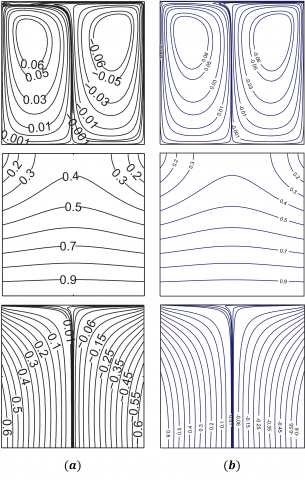Figure 2. Comparison of results by (a). Basak et al.  and (b). Present work

The computations are performed for steady-state flow with the applied maximum relative error of two continuous successive iterations of the order less than $10^{-5}$. In order to validate results obtained by the present FORTRAN code, we compare our numerical solutions with the previous works. Figure 2 illustrates the good comparison of the solutions.

4. Numerical Results

Streamlines for flow behavior, isotherms for temperature contour and heatlines for Cu-water heat transport analysis by the defined boundaries (15)-(17). The physical properties of the water and Cu nanoparticles are listed in Table 1. And also, heat transfer from the walls are specified using $N u$ and $\overline{N u}$. Flow depicted by varying the parameters of $D a=10^{-5}-10^{-1}, R a=10^{3}-10^{5}, \phi=0.05-0.15$ $H a=0-50$ and $P r=6.2$ .

Figure 3 shows the flow responses by changing the Darcy number on $R a=10^{5}, \phi=0.1$ and $H a=5 .$ At $D a=10^{-5}$, a pair of vertically symmetric streamlines flow $\left(\left|\psi_{\max }\right|=0.0035\right)$ is found with inner core circulations at the top side of the cavity. Both the obtained circulations are oppositely oriented. While changing the $D a=10^{-3}$, same flow behavior is visible with increased flow intensity and the shifted inner core circulations of $\left|\psi_{\max }\right|=0.9$ to upper-middle. A secondary pair of circulations near the lower corners of the cavity is depicted while the Darcy number, $D a=10^{-1}$ . Primary circulations are with $\left|\psi_{\max }\right|=7$ found at the center of the cavity. An increased permeability causes the increasing nature of the flow behavior. The inner core primary circulations are shifting towards the middle due to the enhancement in the convection flow. Temperature distribution contours with the parallel and linear variations are obtained at lower Darcy number, $D a=10^{-5}$. A disturbance on parallel temperature distributions are obtained by the increased flow velocities at $D a=10^{-3}$ and intensive temperature distribution with a majority area of $0.6 \leq \theta \leq 0.7$ is noticed at $D a=10^{-1}$. Heatlines $(\Pi)$ in Figure 3 illustrate the heat flow from the bottom wall to the upper portion of the sidewalls. Almost parallelly transferred heatlines from the bottom wall is observed for lower Darcy number and the circulations at the middle portion of the cavity are observed for $D a=10^{-3}$ due to the raise in convective behavior. These heatline circulations are strengthened by changing the $D a$ to $10^{-1}$. By increasing the permeability of the porous medium, the flow velocities are increased due to a decrease in the shear force. The combined action of reduction in the shear force and improvement in the buoyance driven effect leads to an increase in the convection heat transfer.

Effects of Hartmann number at $\phi=0.1, R a=10^{5}$ and $D a=10^{-3}$ are shown in Figure 4. Streamline velocity is found to be reduced from $\left|\psi_{\max }\right|=0.9$ to $\left|\psi_{\max }\right|=0.4$ by updating the $H a=0$ to 25 and further increment of $H a=50$ leads to $\left|\psi_{\max }\right|=0.2$. Here, it is noted that shifting of inner circulations towards the top by increasing the Hartmann number. Also, temperature distribution towards the top of the cavity is reduced and which can be clearly noticed on $0.4 \leq \theta \leq 0.5$. Heatline circulation found while at $H a=0$ gets vanished by increasing the Hartmann number. This vanishing of circulation denotes the reduction of heat transfer, that occurs due to convection. At $H a=50$, heat transfer without any circulations denotes the influence of conduction. Eventually, also spotted unchanged vertically symmetric flows on applying the magnetic field.

## 3.png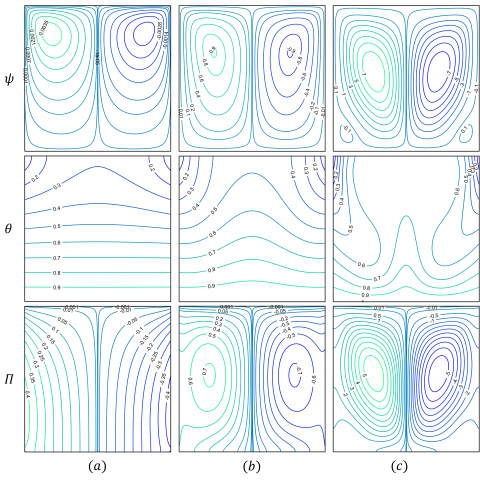Figure 3. Flow patterns for (a). $D a=10^{-5},(b) \cdot D a=10^{-3},(c) \cdot D a=10^{-1}$ at $R a=10^{5}, \phi=0.1$ and $H a=5$

## 4.png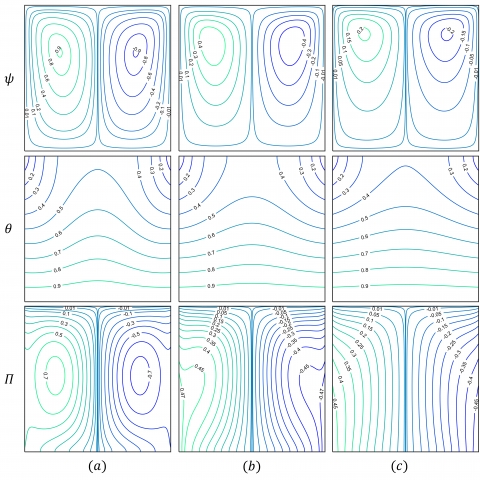Figure 4. Flow patterns for (a). $H a=0,(b) . H a=25,(c) \cdot H a=50$ at $R a=10^{5}, \phi=0.1$ and $D a=10^{-3}$

## 5.pngFigure 5. Flow patterns for (a). $R a=10^{3},(b) \cdot R a=10^{4},(c) \cdot R a=10^{5}$ at $D a=10^{-2}, \phi=0.1$ and $H a=5$

## 6.png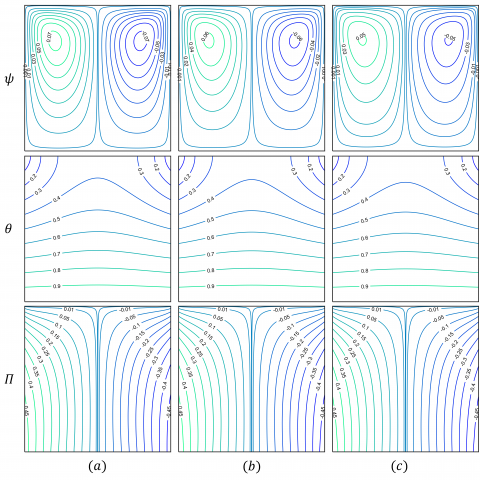Figure 6. Flow patterns for (a) $\phi=0.05,(b) \cdot \phi=0.1,(c) . \phi=0.15$ at $R a=10^{5}, D a=10^{-4}$ and $H a=5$

## 7.png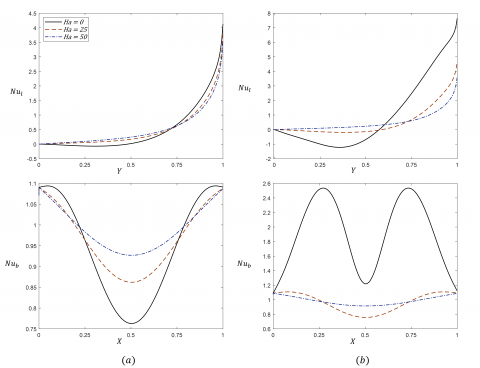Figure 7. Local Nusselt number along the left wall (top) and bottom wall (bottom) at (a) $. D a=10^{-3}$ and $(b) . D a=10^{-1}$

Buoyancy driven effects at $D a=10^{-2}, \phi=0.1$ and $H a=5$ depicted in Figure 5. When the Rayleigh number is low $\left(R a=10^{3}\right)$, natural convective effects are less and thus signifying the lesser circulation intensity on streamlines. The parallel variation on isotherms and heatlines as well as its orthogonally oriented directions indicates the conductive dominated flow over the convection. By increasing the $R a=10^{4}$ , an increase in the intensity of streamline circulations with shifting inner circulations towards the bottom and a disturbance in parallel heatlines flow is observed. Stimulated natural convective effect by changing the $R a$ to $10^{5}$, the velocity of the streamlines gets increased from $\left|\psi_{\max }\right|=0.25$ to $\left|\psi_{\max }\right|=5$. By the given boundary conditions, the secondary pair of circulations are generated due to the increased convective flow. Isotherms are freely distributed by destroying the parallel variation of temperature and increased flow mixing rate. The appearance of heatline circulation and denser heatline at the middle of the cavity for a higher Rayleigh number denotes the rule of convection over the conduction.

Table 1. Physical properties

 Water Cu particle $\rho\left(\mathrm{kg} / \mathrm{m}^{3}\right)$ 997.1 8933 $\mu(P a s)$ $8.9 \times 10^{-4}$ _ $c_{p}(J / k g K)$ 4179 385 $k(W / m K)$ 0.613 401 $\beta\left(K^{-1}\right)$ $2.1 \times 10^{-4}$ $16.7 \times 10^{-4}$

Figure 6 shows up the changes in flow fields by varying the nanoparticle solid volume fraction $(\phi=0.05,0.1,0.15)$. An increase in the solid volume fraction of the Cu nanoparticles decreases the streamlines velocity as $\left|\psi_{\max }\right|=0.07$ for $\phi=0.05,\left|\psi_{\max }\right|=0.06$ for $\phi=0.1$ and $\left|\psi_{\max }\right|=0.05$ for $\phi=0.15 .$. This suppression of velocities on Streamfunction is due to the fact that, increase in viscosity of the running fluid by the addition of Cu nanoparticles. These added nanoparticles involve in the opposition of flow velocities. The minor change in heatlines is observed near the bottom sides $(\Pi=0.45)$ by the enhanced nature of heat transfer.

Heat transfer along the distance of the left wall and bottom wall are shown in Figure 7. In the left wall, local Nusselt number increases with the $Y=0$ to $Y=1$. Minima $N u_{l}$ found in between $0.25 \leq Y \leq 0.5$ at $H a=0$ and minima increases with the increase in Ha. In vise versa, maxima of $N u_{l}$ decreases with an increase in Ha. At $D a=10^{-1}$(Figure 7b), increase in $N u_{l}$ observed by increased convective effect by strengthening the permeability. On the bottom wall, $N u_{b}$ increases near both the sidewalls and decreases in the middle. While increasing the Hartmann number, the sinusoidal variations are interrupted and smooth variations on $N u_{b}$ is noted.

Figure 8 represents the average Nusselt number along the left wall and bottom walls respectively. Same heat transfer effects are noted on both the left wall and right wall, which can be clearly cited by the similarity in the heatlines on both the vertical half of the cavity. Figure 8a illustrates the effect of $H a$ and $D a$ on $\overline{N u_{l}}$ and $\overline{N u_{b}}$. Here the decrease in convective heat transfer is noted, which effects are more visible at the higher Da. Figure 8b signifies the nanoparticles $(\phi)$ advancement of heat transfer effect. Here, an increase in Ra increases the convection effects and causes increased convective heat transfer. In general, the constantly heated bottom wall delivers more heat transfer than the linearly heated left wall.

## 8.png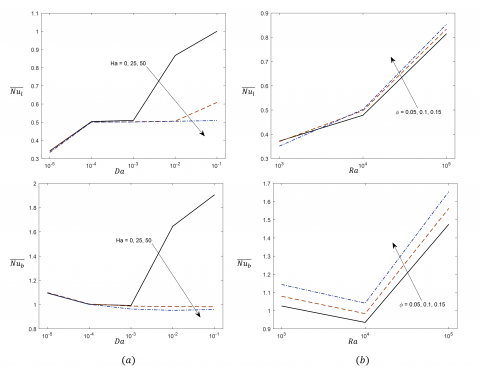Figure 8. Average Nusselt number vs left wall (top) and bottom wall (bottom) at $(a) \cdot R a=10^{5}, \phi=0.1$ and $(b) \cdot H a=5, D a=10^{-2}$

5. Conclusions

The investigation was carried out based on a linearly decreasing temperature boundary towards the upper portion of the porous cavity. The study considers nano-sized particles dispersed in the base fluid, in order to enhance the thermal conductivity of the conventional base fluids. The Cu-water nanofluid flows due to the MHD natural convection in the cavity illustrates the following main results,

• Nanofluid streamline flow on a higher Darcy number and a higher Rayleigh number produces stronger circulation on the primary core and is involved in the generation of the secondary pair of circulations.
• An increase in viscosity of the nanofluid by increasing the concentration of the nanoparticles volume fraction causes a reduction in the streamlines velocity but increases the heat transfer rate.
• The convection heat transfer is improved for higher values of the Darcy number and Rayleigh number. But augment in the convection heat transfer is noticed for increasing the strength of an applied magnetic field.
• An increase in Hartmann number suppresses the heatline circulation and leads to orthogonal heatlines against the isotherm contour signifies the action of conduction heat transfer. Thus, by quantifying the effect of an applied magnetic field, the domination of convection heat transfer gets suppressed.

Some future research interest based on the authors' suggestion is,

• Further studies can be carried out based on different geometries and various other combinations of nanofluids can be considered.
• To improve the stability and thermal conductivity of the nanofluids, a different combination of hybrid nanofluids can be incorporated into the study.
• A detailed study on entropy analysis in a porous cavity filled with the various nanofluids can be considered.
• Transient state flows and turbulent natured flows can be incorporated into the study. Since lesser research is carried out on turbulent flows.
Acknowledgment

This work is supported by partial financial support through TEQIP-III Project, NIT Arunachal Pradesh and support through DST project, Sanction number: SERB ECR/2017/001007.

References

 Kaviany, M. (1995). Principles of Heat Transfer in Porous Media. Springer, New York. https://doi.org/10.1007/978-1-4612-4254-3

 Bejan, A. (2006). Convection in Porous Media. In Convection in Porous Media. https://doi.org/10.1007/0-387-33431-9

 Khanafer, K., Vafai, K. (2019). Applications of nanofluids in porous medium: A critical review. Journal of Thermal Analysis and Calorimetry, 135(2): 1479-1492. https://doi.org/10.1007/s10973-018-7565-4

 Oztop, H.F., Abu-Nada, E. (2008). Numerical study of natural convection in partially heated rectangular enclosures filled with nanofluids. International Journal of Heat and Fluid Flow, 29(5): 1326-1336. https://doi.org/10.1016/j.ijheatfluidflow.2008.04.009

 Sathiyamoorthy, M., Chamkha, A. (2010). Effect of magnetic field on natural convection flow in a liquid gallium filled square cavity for linearly heated side wall(s). International Journal of Thermal Sciences, 49(9): 1856-1865. https://doi.org/https://doi.org/10.1016/j.ijthermalsci.2010.04.014

 Sivasankaran, S., Malleswaran, A., Lee, J., Sundar, P. (2011). Hydro-magnetic combined convection in a lid-driven cavity with sinusoidal boundary conditions on both sidewalls. International Journal of Heat and Mass Transfer, 54(1-3): 512-525. https://doi.org/10.1016/j.ijheatmasstransfer.2010.09.018

 Al-Salem, K., Öztop, H. F., Pop, I., Varol, Y. (2012). Effects of moving lid direction on MHD mixed convection in a linearly heated cavity. International Journal of Heat and Mass Transfer, 55(4): 1103-1112. https://doi.org/10.1016/j.ijheatmasstransfer.2011.09.062

 Nasrin, R., Alim, M.A. (2012). Soret and Dufour effects on double diffusive natural convection in a chamber utilizing nanofluid. International Journal of Heat and Technology, 30(1): 109-117.

 Sathiyamoorthy, M., Chamkha, A.J. (2014). Analysis of natural convection in a square cavity with a thin partition for linearly heated side walls. International Journal of Numerical Methods for Heat and Fluid Flow, 24(5): 1057-1072. https://doi.org/10.1108/HFF-02-2012-0050

 Ahrara, A.J., Djavareshkianb, M.H., Ataiyanc, M. (2017). Numerical simulation of Cu-water nanofluid magneto-hydro-dynamics and heat transfer in a cavity containing a circular cylinder of different size and positions. International Journal of Heat and Technology, 35(2): 403-415. https://doi.org/10.18280/ijht.350225

 Mahmoodi, M., Abbasian Arani, A.A., Mazrouei Sebdani, S., Tajik, P. (2017). Natural convection in nanofluid-filled square chambers subjected to linear heating on both sides: A numerical study. Heat Transfer Research, 48(9): 771-785. https://doi.org/10.1615/HeatTransRes.2016011010

 Tayebi, T., Chamkha, A.J. (2017). Buoyancy-driven heat transfer enhancement in a sinusoidally heated enclosure utilizing hybrid nanofluid. Computational Thermal Sciences, 9(5): 405-421. https://doi.org/10.1615/ComputThermalScien.2017019908

 Alam, M.F., Bora, M.K., Sharma, B., Barman, R.N. (2019). Numerical investigation of magneto-hydrodynamics mixed convection in a square cavity for various shaped conducting obstacles placed at the center. Mathematical Modelling of Engineering Problems, 6(4): 550-556. https://doi.org/10.18280/mmep.060410

 Triveni, M.K., Panua, R. (2019). Free convection in a caterpillar shaped triangular enclosure filled with different nanofluids. International Journal of Heat and Technology, 37(2): 398-406. https://doi.org/10.18280/ijht.370204

 Chaabane, R., Jemni, A. (2020). On the numerical treatment of magneto-hydro dynamics free convection with mixed boundary conditions. Mathematical Modelling of Engineering Problems, 7(3): 421-426. https://doi.org/10.18280/mmep.070312

 Giwa, S.O., Sharifpur, M., Ahmadi, M.H., Meyer, J.P. (2020). A review of magnetic field influence on natural convection heat transfer performance of nanofluids in square cavities. In Journal of Thermal Analysis and Calorimetry (Issue 0123456789). Springer International Publishing. https://doi.org/10.1007/s10973-020-09832-3

 Al-Kouz, W., Saleem, K.B., Chamkha, A. (2020). Numerical investigation of rarefied gaseous flows in an oblique wavy sided walls square cavity. International Communications in Heat and Mass Transfer, 116, 104719. https://doi.org/10.1016/j.icheatmasstransfer.2020.104719

 Oztop, H.F., Mobedi, M., Abu-Nada, E., Pop, I. (2012). A heatline analysis of natural convection in a square inclined enclosure filled with a CuO nanofluid under non-uniform wall heating condition. International Journal of Heat and Mass Transfer, 55(19-20): 5076-5086. https://doi.org/10.1016/j.ijheatmasstransfer.2012.05.007

 Ahmed, K.F.U., Parvin, S., Chamkha, A.J. (2015). Numerical analysis based on heatline approach for natural convection flows within prismatic enclosures. International Journal of Energy & Technology, 7: 19-29.

 Alam, M.S., Rahman, M.M., Parvin, S., Vajravelu, K. (2016). Finite element simulation for heatline visualization of natural convective flow and heat transfer inside a prismatic enclosure. International Journal of Heat and Technology, 34(3): 391-400. https://doi.org/10.18280/ijht.340307

 Alsabery, A.I., Chamkha, A.J., Saleh, H., Hashim, I. (2016). Heatline visualization of conjugate natural convection in a square cavity filled with nanofluid with sinusoidal temperature variations on both horizontal walls. International Journal of Heat and Mass Transfer, 100: 835-850. https://doi.org/10.1016/j.ijheatmasstransfer.2016.05.031

 Walker, K.L., Homsy, G.M. (1978). Convection in a porous cavity. Journal of Fluid Mechanics, 87(3): 449-474. https://doi.org/10.1017/S0022112078001718

 Prasad, V., Kulacki, F.A. (1984). Corrective heat transfer in a rectangular porous cavity-effect of aspect ratio on flow structure and heat transfer. Journal of Heat Transfer, 106(1): 158-165. https://doi.org/10.1115/1.3246629

 Mahmud, S., Fraser, R.A. (2004). Magnetohydrodynamic free convection and entropy generation in a square porous cavity. International Journal of Heat and Mass Transfer, 47(14-16): 3245-3256. https://doi.org/10.1016/j.ijheatmasstransfer.2004.02.005

 Sathiyamoorthy, M., Basak, T., Roy, S., Pop, I. (2007). Steady natural convection flow in a square cavity filled with a porous medium for linearly heated side wall(s). International Journal of Heat and Mass Transfer, 50(9-10): 1892–1901. https://doi.org/10.1016/j.ijheatmasstransfer.2006.10.010

 Varol, Y., Oztop, H.F., Pop, I. (2008). Numerical analysis of natural convection for a porous rectangular enclosure with sinusoidally varying temperature profile on the bottom wall. International Communications in Heat and Mass Transfer, 35(1): 56-64. https://doi.org/10.1016/j.icheatmasstransfer.2007.05.015

 Basak, T., Krishna Pradeep, P.V., Roy, S., Pop, I. (2011). Finite element based heatline approach to study mixed convection in a porous square cavity with various wall thermal boundary conditions. International Journal of Heat and Mass Transfer, 54(9-10): 1706-1727. https://doi.org/10.1016/j.ijheatmasstransfer.2010.12.043

 Ramakrishna, D., Basak, T., Roy, S., Pop, I. (2013). Analysis of heatlines during natural convection within porous square enclosures: Effects of thermal aspect ratio and thermal boundary conditions. International Journal of Heat and Mass Transfer, 59(1): 206-218. https://doi.org/10.1016/j.ijheatmasstransfer.2012.11.076

 Kasaeian, A., Azarian, R.D., Mahian, O., Kolsi, L., Chamkha, A.J., Wongwises, S., Pop, I. (2017). Nanofluid flow and heat transfer in porous media: A review of the latest developments. In International Journal of Heat and Mass Transfer, 107: 778-791. https://doi.org/10.1016/j.ijheatmasstransfer.2016.11.074

 Abdulkadhim, A., Abed, A.M., Mohsen, A.M., Al-Farhany, K. (2018). Effect of partially thermally active wall on natural convection in porous enclosure. Mathematical Modelling of Engineering Problems, 5(4): 395-406. https://doi.org/10.18280/mmep.050417

 Al-Srayyih, B.M., Gao, S., Hussain, S.H. (2019). Effects of linearly heated left wall on natural convection within a superposed cavity filled with composite nanofluid-porous layers. Advanced Powder Technology, 30(1): 55-72. https://doi.org/10.1016/j.apt.2018.10.007

 Kumar, A.V., Jino, L., Berlin, M., Mohanty, P.K. (2019). Magnetic field effect on nanofluid suspension cavity by non-uniform boundary conditions. AIP Conference Proceedings, 2134. https://doi.org/10.1063/1.5120205

 Belhadj, M., Atia, A., Benchatti, A. (2020). Analysis of natural convection in porous media for thermal storage using Darcy-Brinkman-Forcheimer formulation. Mathematical Modelling of Engineering Problems, 7(1): 73-78. https://doi.org/10.18280/mmep.070109

 Bouafia, I., Mehdaoui, R., Kadri, S., Elmir, M. (2020). Natural convection in a porous cavity filled with nanofluid in the presence of isothermal corrugated source. International Journal of Heat and Technology, 38(2): 334-342. https://doi.org/10.18280/ijht.380208

 Jino, L., Vanav Kumar, A. (2020). Cu-water nanofluid natural convective heat and fluid flow in a porous cavity. International Journal of Mechanical and Production Engineering Research and Development, 10(3): 13695-13706. https://doi.org/10.24247/ijmperdjun20201305

 Jino, L., Vanav Kumar, A. (2020). Natural convection of water-cu nanofluid in a porous cavity with two pairs of heat source-sink and magnetic effect. International Journal of Mechanical and Production Engineering Research and Development, 10(3): 14481-14492. https://doi.org/10.24247/ijmperdjun20201378

 Wilkes, J.O., Churchill, S.W. (1966). The finite‐difference computation of natural convection in a rectangular enclosure. AIChE J., 12(1): 161-166. https://doi.org/10.1002/aic.690120129